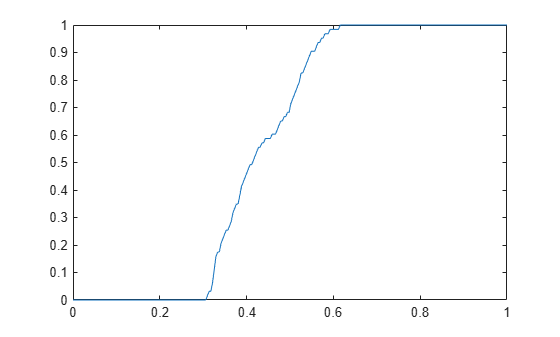## Histogram Equalization

The process of adjusting intensity values can be done automatically using histogram equalization. Histogram equalization involves transforming the intensity values so that the histogram of the output image approximately matches a specified histogram. By default, the histogram equalization function, `histeq`, tries to match a flat histogram with 64 bins, but you can specify a different histogram instead.

Notice how this curve reflects the histograms in the previous figure, with the input values mostly between 0.3 and 0.6, while the output values are distributed evenly between 0 and 1.

### Adjust Intensity Values Using Histogram Equalization

This example shows how to use histogram equalization to adjust the contrast of a grayscale image. The original image has low contrast, with most pixel values in the middle of the intensity range. `histeq` produces an output image with pixel values evenly distributed throughout the range.

Read an image into the workspace.

`I = imread('pout.tif');`

Display the image and its histogram.

```figure subplot(1,2,1) imshow(I) subplot(1,2,2) imhist(I,64)```Adjust the contrast using histogram equalization. In this example, the histogram equalization function, `histeq`, tries to match a flat histogram with 64 bins, which is the default behavior. You can specify a different histogram instead.

`J = histeq(I);`

Display the contrast-adjusted image and its new histogram.

```figure subplot(1,2,1) imshow(J) subplot(1,2,2) imhist(J,64)```### Plot Transformation Curve for Histogram Equalization

This example shows how to plot the transformation curve for histogram equalization. `histeq` can return a 1-by-256 vector that shows, for each possible input value, the resulting output value. (The values in this vector are in the range [0,1], regardless of the class of the input image.) You can plot this data to get the transformation curve.

`I = imread('pout.tif');`

Adjust the contrast using histogram equalization, using the `histeq` function. Specify the gray scale transformation return value, `T`, which is a vector that maps graylevels in the intensity image `I` to gray levels in `J`.

`[J,T] = histeq(I);`

Plot the transformation curve. Notice how this curve reflects the histograms in the previous figure, with the input values mostly between 0.3 and 0.6, while the output values are distributed evenly between 0 and 1.

```figure plot((0:255)/255,T);```